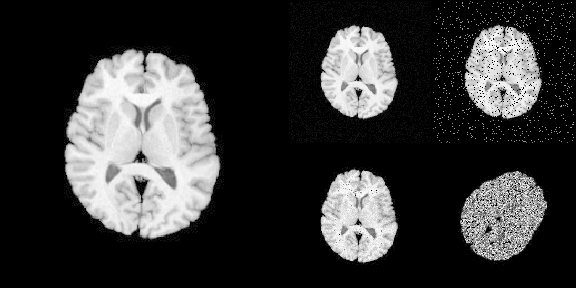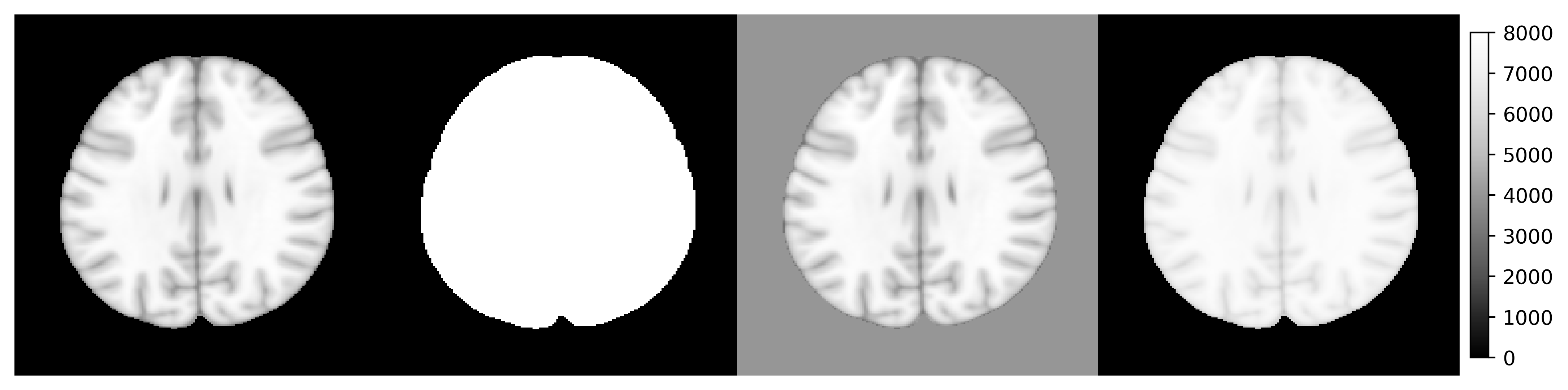rpyANTs was detached from a RAVE (Reproducible Analysis and Visualization of iEEG) module. It is now a standalone package that connects ANTsPy with R using seamless shared-memory.

This package was originally created for the following three purposes:

• Portability
• Make ANTs easily accessible from the latest R and all major operating systems
• Allow RAVE or other code/scripts/frameworks to be reproducible since the code will be OS-invariant
• Easy to install
• Automated installation that requires very little to no knowledge about compilers
• Installing rpyANTs takes less than 10 minutes
• The goal is to have minimum human intervention
• Easy to embed
• Python scripts using ANTsPy can be executed from rpyANTs and R with no modification
• Built-in bilateral data conversions between Python and R allows image generated from Python to be analyzed/visualized in R and vice versa

Disclaimer: This is a third-party maintained R package for ANTs. If you are looking for the ANTsR package by B.B Avants, please check here.

## Installation

The installation requires one-line extra setup

# Install from CRAN
install.packages("rpyANTs")

# Install from nightly dev builder
# install.packages("rpyANTs", repos = "https://dipterix.r-universe.dev")

# set up ANTs
rpyANTs::install_ants()

install_ants creates an isolated Python environment managed by RAVE. This environment does not conflict nor affect your existing Python installations.

### Upgrade ANTs

To upgrade ANTs, first update rpyANTs, then upgrade ANTsPyx

install.packages("rpyANTs")
rpymat::add_packages(packages = "antspyx", pip = TRUE)

## How to use

To load ANTs

library(rpyANTs)

# Whether ANTs is available
ants_available()

ants

In R, we use $ to get module functions or class members. For example: ants$add_noise_to_image
#> <ANTs Python Wrapper>
#>
#>     shot, or speckle noise.
#>
#>     ANTsR function: addNoiseToImage
#>
#>     Arguments
#>     ---------
#>     image : ANTsImage
#>         scalar image.
#>
#>     noise_model : string
#>         'additivegaussian', 'saltandpepper', 'shot', or 'speckle'.
#>
#>     noise_parameters : tuple or array or float
#>         'saltandpepper': (probability, saltValue, pepperValue)
#>         'shot': scale
#>         'speckle': standardDeviation
#>
#>     Returns
#>     -------
#>     ANTsImage
#>
#>     Example
#>     -------
#>     >>> import ants
#>     >>> noise_image = ants.add_noise_to_image(image, 'saltandpepper', (0.1, 0.0, 100.0))
#>     >>> noise_image = ants.add_noise_to_image(image, 'shot', 1.0)
#>     >>> noise_image = ants.add_noise_to_image(image, 'speckle', 1.0)
#>
#> *** Above documentation is for Python.
#> *** Please use $ instead of . for modules and functions in R #> <function add_noise_to_image at 0x113867060> The following R code translates Python code into R: # >>> img = ants.image_read(ants.get_ants_data('r16')) img <- ants$image_read(ants$get_ants_data('r16')) # >>> noise_image1 = ants.add_noise_to_image(img, 'additivegaussian', (0.0, 1.0)) noise_image1 <- ants$add_noise_to_image(
noise_parameters = tuple(0.0, 1.0)
)

# >>> noise_image2 = ants.add_noise_to_image(img, 'saltandpepper', (0.1, 0.0, 100.0))
noise_image2 <- ants$add_noise_to_image( img, 'saltandpepper', noise_parameters = tuple(0.1, 0.0, 100.0) ) # >>> noise_image3 = ants.add_noise_to_image(img, 'shot', 1.0) noise_image3 <- ants$add_noise_to_image(
img, 'shot',
noise_parameters = 1.0
)

# >>> noise_image4 = ants.add_noise_to_image(img, 'speckle', 1.0)
noise_image4 <- ants$add_noise_to_image( img, 'speckle', noise_parameters = 1.0 ) # >>> trans = ants.create_ants_transform( # >>> dimension=2, matrix=[[0.707, 0.707], [-.707, 0.707]], # >>> translation=[-53, 128]) trans <- as_ANTsTransform(matrix( c(0.707, 0.707, -53, -0.707, 0.707, 128), nrow = 2, byrow = TRUE ), dimension = 2) # >>> noise_image4 = trans.apply_to_image(noise_image4) noise_image4 <- trans$apply_to_image(noise_image4)

To load imaging data into R

# Use [] to convert ANTsImage into R array
is.array(img[])
#>  TRUE

# plot via R
layout(matrix(c(1,1,2,3,1,1,4,5), nrow = 2, byrow = TRUE))
par(mar = c(0.1, 0.1, 0.1, 0.1), bg = "black", fg = "white")
pal <- grDevices::gray.colors(256, start = 0, end = 1)

image(img[], asp = 1, axes = FALSE,
col = pal, zlim = c(0, 255), ylim = c(1, 0))
image(noise_image1[], asp = 1, axes = FALSE,
col = pal, zlim = c(0, 255), ylim = c(1, 0))
image(noise_image2[], asp = 1, axes = FALSE,
col = pal, zlim = c(0, 255), ylim = c(1, 0))
image(noise_image3[], asp = 1, axes = FALSE,
col = pal, zlim = c(0, 255), ylim = c(1, 0))
image(noise_image4[], asp = 1, axes = FALSE,
col = pal, zlim = c(0, 255), ylim = c(1, 0))### Run/Debug Python scripts

rpyANTs ports functions that allows to run Python scripts. For example:

library(rpyANTs)

script_path <- tempfile(fileext = ".py")
writeLines(con = script_path, text = r"(

# This is Python script
import ants
print(ants.__version__)

)")

run_script(script_path)

You can also run Python interactive in R (yes, you are correct). Simply run

rpyANTs::repl_python()

The console prefix will change from > to >>>, meaning you are in Python mode:

> rpyANTs::repl_python()
Python 3.8.16 (/Users/dipterix/Library/r-rpymat/miniconda/envs/rpymat-conda-env/bin/python3.8)
Reticulate 1.26 REPL -- A Python interpreter in R.
Enter 'exit' or 'quit' to exit the REPL and return to R.
>>> 

Try some Python code!

>>> import ants
>>> help(ants.registration)

To exit Python mode, type exit (no parenthesis) and hit enter key

>>> exit
> 

### Data conversions

Native R variables can be easily converted to Python and back via r_to_py and py_to_r.

For example

# R to Python
r_to_py(1)
#> 1.0
r_to_py(1L)
#> 1

# Python to R
py_obj <- py_list(1:3)
class(py_obj)  # <- this is a python object
#>  "python.builtin.list"   "python.builtin.object"

py_to_r(py_obj)
#>  1 2 3

You can also use variables created in R from Python or vice versa:

In the following example, an R object object_r is created. In Python, it can be accessed (read-only) via r.object_r

> object_r <- c(1,2,3)
> repl_python()
Python 3.8.16 (/Users/dipterix/Library/r-rpymat/miniconda/envs/rpymat-conda-env/bin/python3.8)
Reticulate 1.26 REPL -- A Python interpreter in R.
Enter 'exit' or 'quit' to exit the REPL and return to R.
>>> r.object_r
[1.0, 2.0, 3.0]

Similarly, a Python object object_py is created, and it can be read from py$object_py: >>> import numpy as np >>> object_py = np.array([2,3,4]) >>> exit > py$object_py
 2 3 4

## Known issues

### Variable types

R is not a type-rigid language. Some functions in ANTsPy require specific variable types that are often vague in R. For example the dimension argument in function ants$create_ants_transform needs to be an integer, but R’s default numerical values are double. In this case, variable formats need to be explicitly given. Here are several examples 1. Explicit integers # ants$create_ants_transform(dimension = 3)     # <- error
ants$create_ants_transform(dimension = 3L) # < XXXL is an explicit integer 1. Tuple, list, and dictionary A Python tuple is a vector that cannot alter lengths. # Wrong as aff_iterations needs to be a tuple # ants$registration(fixed, moving, ..., aff_iterations = c(6L, 4L, 2L, 1L))

ants$registration(fixed, moving, ..., aff_iterations = tuple(6L, 4L, 2L, 1L)) Similar conversions can be done via py_list, py_dict. 1. Convert TRUE vs. FALSE A Python module can be imported with auto-conversion (argument convert) set to TRUE or FALSE. When auto-conversion is on, the Python function results will be converted to R objects automatically. For example, np <- import("numpy", convert = TRUE) np$eye(4L)
#>      [,1] [,2] [,3] [,4]
#> [1,]    1    0    0    0
#> [2,]    0    1    0    0
#> [3,]    0    0    1    0
#> [4,]    0    0    0    1

The numpy array is automatically translated as an R matrix. While this is convenient, this automated conversion could cause some issues when the function results are further passed into another Python function. For example, the following code will raise errors.

> np <- import("numpy", convert = TRUE)
>
> image <- ants$image_read(ants$get_ants_data('mni'))
> image_array <- np$asarray(list(image, image)) > > ants$plot_grid(image_array, slices = 100L)

Error in py_call_impl(callable, dots$args, dots$keywords) :
Matrix type cannot be converted to python (only integer, numeric, complex, logical, and character matrixes can be converted

The error is raised because numpy has convert=TRUE, hence image_array is converted to an R list with each element being a ANTsImage instance. Calling ants$plot_grid needs R-to-Python conversion for all input variables, including image_array. However this conversion makes image_array a Python list instead of numpy array, violating the input format. A safer way is to keep in the Python format, i.e. convert=FALSE. In this mode, function results will not be converted back to R (you need to manually make conversion by yourself via py_to_r). Now the following example works. > np <- import("numpy", convert = TRUE) > ants <- load_ants() > > image <- ants$image_read(ants$get_ants_data('mni')) > image_array <- np$asarray(list(image, image))
>
> ants$plot_grid(image_array, slices = 100L) Object ants in rpyANTs is a non-conversion Python module. Object py is a auto-conversion Python module ### Operators In Python, operators on ANTsImage, such as img > 5 are defined. Such operators is being supported in R as S3 generic functions. Don’t worry if you don’t know what is S3 generic, see the following examples: library(rpyANTs) image <- ants$image_read(ants$get_ants_data('mni')) print(image) dim(image) range(image) y1 <- (image > 10) * 8000 y2 <- image y2[y2 < 10] <- 4000 y3 <- log(image + 1000) y3 <- (y3 - min(y3)) / (max(y3) - min(y3)) * 8000 ants_plot_grid( list(image, y1, y2, y3), slices = 100, shape = c(1, 4), vmin = 0, vmax = 8000 )Although the operator generics have been implemented for common classes such as ANTsImage and ANTsTransform. Many are still under development and not supported. In this case, you might want to use the following workaround methods. You are more than welcome to post a wish-list or issue ticket to the Github repository Alternative version 1: call operators directly library(rpyANTs) image <- ants$image_read(ants$get_ants_data('r16')) # The followings are the same # threshold <- image > 10 threshold <- image$__gt__(10)
ants$plot(threshold) Work-around version 2: If you don’t know how Python operators work, use Python directly library(rpyANTs) image <- ants$image_read(ants$get_ants_data('r16')) # Create an R variable from Python! py_run_string("r.threshold = r.image > 10", local = TRUE, convert = FALSE) ants$plot(threshold)

## Citation

This is a general citation for ANTs:

Avants, B.B., Tustison, N. and Song, G., 2009. Advanced normalization tools (ANTS). The Insight Journal, 2(365), pp.1-35.

If you are using rpyANTs through RAVE or YAEL, please also cite:

Magnotti, J.F., Wang, Z. and Beauchamp, M.S., 2020. RAVE: Comprehensive open-source software for reproducible analysis and visualization of intracranial EEG data. NeuroImage, 223, p.117341.

This package rpyANTs is released under Apache-2.0 license (Copyright: Zhengjia Wang). The underlying ANTsPy is released under Apache-2.0 license (Copyright: ANTs contributors).